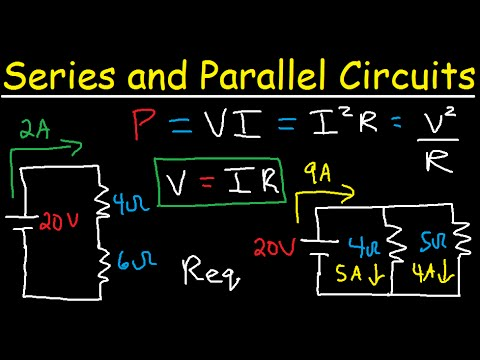What is the difference between series and parallel circuits

# What is the difference between series and parallel circuitsWhat is the difference between series and parallel circuits

Components of an electrical circuit or electronic circuit can be connected in series, parallel, or series-parallel. The two simplest of these are called series and parallel and occur frequently. Components connected in series are connected along a single conductive path, so the same current flows through all of the components but voltage is dropped (lost) across each of the resistances.
In a series circuit, the sum of the voltages consumed by each individual resistance is equal to the source voltage. Components connected in parallel are connected along multiple paths so that the current can split up; the same voltage is applied to each component.

A circuit composed solely of components connected in series is known as a series circuit; likewise, one connected completely in parallel is known as a parallel circuit.

In a series circuit, the current that flows through each of the components is the same, and the voltage across the circuit is the sum of the individual voltage drops across each component. In a parallel circuit, the voltage across each of the components is the same, and the total current is the sum of the currents flowing through each component.

Consider a very simple circuit consisting of four light bulbs and a 12-volt automotive battery. If a wire joins the battery to one bulb, to the next bulb, to the next bulb, to the next bulb, then back to the battery in one continuous loop, the bulbs are said to be in series. If each bulb is wired to the battery in a separate loop, the bulbs are said to be in parallel.

If the four light bulbs are connected in series, the same current flows through all of them and the voltage drop is 3-volts across each bulb, which may not be sufficient to make them glow. If the light bulbs are connected in parallel, the currents through the light bulbs combine to form the current in the battery, while the voltage drop is 12-volts across each bulb and they all glow.

In a series circuit, every device must function for the circuit to be complete. If one bulb burns out in a series circuit, the entire circuit is broken. In parallel circuits, each light bulb has its own circuit, so all but one light could be burned out, and the last one will still function.

This physics video tutorial explains series and parallel circuits.  It contains plenty of examples, equations, formulas, and practice problems showing you how to solve it with all of the necessary calculations.

Related Topics:

Continue Reading about the "What is the difference between series and parallel circuits" on the next page below